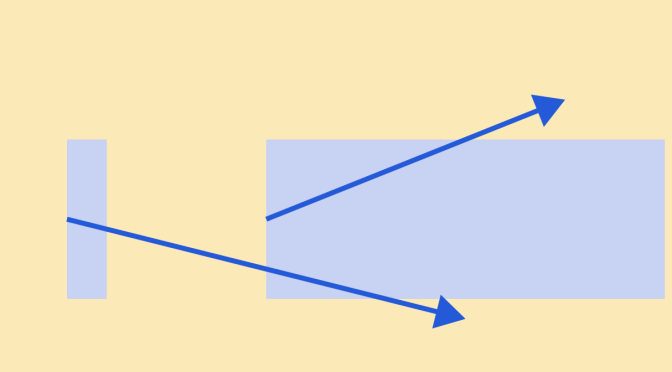# Counterexamples around balls in metric spaces

Let’s play with balls in a metric space $$(M,d)$$. We denote by

• $$B_r(p) = \{x \in M : d(x,p) < r\}$$ the open ball.
• $$B_r[p] = \{x \in M : d(x,p) \le r\}$$ the closed ball.

### A ball of radius $$r$$ included in a ball of radius $$r^\prime < r$$

We take for $$M$$ the space $$\{0\} \cup [2, \infty)$$ equipped with the standard metric distance $$d(x,y)=\vert x – y \vert$$.

We have $$B_4(0) = \{0\} \cup [2, 4)$$ while $$B_3(2) = \{0\} \cup [2, 5)$$. Despite having a strictly smaller radius, the ball $$B_3(2)$$ strictly contains the ball $$B_4(0)$$.

The phenomenon cannot happen in a normed vector space $$(M, \Vert \cdot \Vert)$$. For the proof, take two open balls $$B_r(p),B_{r^\prime}(p^\prime) \subset M$$, $$0 < r^\prime < r$$ and suppose that $$p \in B_{r^\prime}(p^\prime)$$. If $$p=p^\prime$$ and $$q \in B_{r^\prime}(p^\prime) \setminus \{p^\prime\}$$ then $$p + \frac{\frac{r+r^\prime}{2} }{\Vert p q \Vert} p q \in B_r(p) \setminus B_{r^\prime}(p^\prime)$$. And if $$p \neq p^\prime$$, $$p \in B_{r^\prime}(p^\prime)$$ then $$p^\prime + \frac{\frac{r+r^\prime}{2} }{\Vert p^\prime p \Vert} p^\prime p \in B_r(p) \setminus B_{r^\prime}(p^\prime)$$.

### An open ball $$B_r(p)$$ whose closure is not equal to the closed ball $$B_r[p]$$

Here we take for $$M$$ a subspace of $$\mathbb R^2$$ which is the union of the origin $$\{0\}$$ with the unit circle $$S^1$$. For the distance, we use the Euclidean norm.
The open unit ball centered at the origin $$B_1(0)$$ is reduced to the origin: $$B_1(0) = \{0\}$$. Its closure $$\overline{B_1(0)}$$ is itself. However the closed ball $$B_1$$ is the all space $$\{0\} \cup S^1$$.

Again one can prove that for a normed vector space this cannot happen. The closure of an open ball is the closed ball for a normed vector space.